﻿ 基于特征空间匹配的激光雷达点云配准算法文章快速检索 高级检索
 大地测量与地球动力学2020, Vol. 40Issue (12): 1303-1307  DOI: 10.14075/j.jgg.2020.12.019### 引用本文CHEN Qiang, YUE Dongjie, CHEN Jian. Laser LiDAR Point Cloud Registration Algorithm Based on Feature Space Matching[J]. Journal of Geodesy and Geodynamics, 2020, 40(12): 1303-1307.### About the first author

CHEN Qiang, postgraduate, majors in 3D laser scanning point cloud data processing, E-mail:1182695648@qq.com.

### 文章历史

1. 河海大学地球科学与工程学院，南京市佛城西路8号，211100

1 基于特征空间匹配的配准算法

1.1 ISS特征点提取

1)为点云中每个点pi建立局部坐标系，设定每个点的搜索半径r

2)以搜索半径r建立k-d树，计算其范围内所有点的权值：

 ${\mathit{\boldsymbol{w}}_{ij}} = 1/\left| {{\mathit{\boldsymbol{p}}_i} - {\mathit{\boldsymbol{p}}_j}} \right|, \left| {{\mathit{\boldsymbol{p}}_i} - {\mathit{\boldsymbol{p}}_j}} \right| < r$ (1)

3)计算所有点pi的协方差矩阵：

 $\begin{array}{*{20}{c}} {{\mathop{\rm cov}} \left( {{\mathit{\boldsymbol{p}}_i}} \right) = }\\ {\sum\limits_{\left| {{\mathit{\boldsymbol{p}}_i} - {\mathit{\boldsymbol{p}}_j}} \right| < r} {{\mathit{\boldsymbol{w}}_{ij}}} \left( {{\mathit{\boldsymbol{p}}_i} - {\mathit{\boldsymbol{p}}_j}} \right){{\left( {{\mathit{\boldsymbol{p}}_i} - {\mathit{\boldsymbol{p}}_j}} \right)}^{\rm{T}}}/\sum\limits_{\left| {{\mathit{\boldsymbol{p}}_i} - {\mathit{\boldsymbol{p}}_j}} \right| < r} {{\mathit{\boldsymbol{w}}_{ij}}} } \end{array}$ (2)

4)计算各点pi协方差矩阵的特征值{λi1λi2λi3}，由大到小进行排列。

5)设置阈值ε1ε2，满足式(3)则认为该点为ISS特征点：

 $\lambda _i^2/\lambda _i^1 \le {\varepsilon _1}, \lambda _i^3/\lambda _i^2 \le {\varepsilon _2}$ (3)
1.2 改进的PointNet模型

PointNet为一种新型的处理点云数据的深度学习模型，可直接在点云数据上应用。该模型在点云分类和任务分割中具有重要作用，但所获取的点云特征未充分考虑邻域点的信息，因此难以直接应用于点云配准。图 1为PointNet主要架构，关键流程包括：输入为点云数据的坐标集合；通过网络得到转换矩阵，将原始点云与转换矩阵相乘进行点云对齐；再通过多层感知器(multilayer perceptron, MLP)提取点云数据特征，对特征进行对齐并升维；执行最大池化操作来获取各组点云数据的全局特征；最后针对点云分类或任务分割，分别对特征进行MLP处理。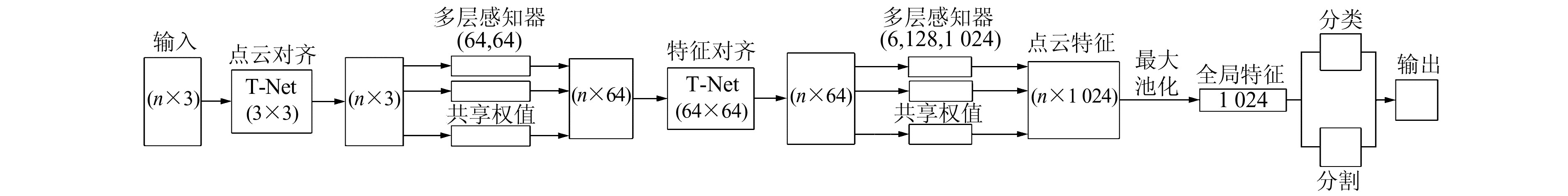图 1 PointNet简略架构图 Fig. 1 Simple architecture diagram of PointNet

PointNet处理点云数据具有可提取深度信息的优点，但其在点云配准上仍具有一定挑战：PointNet对于姿态错位较为敏感，旋转不变性的前提是点云相对于规范坐标框架错位较小；需要大量的训练数据来提高抗噪性；原始模型针对不同样本需要等量点数等。

 ${\mathit{\boldsymbol{F}}_{12}} = \left( {{{\left\| \mathit{\boldsymbol{d}} \right\|}_2}, \angle \left( {{\mathit{\boldsymbol{n}}_1}, \mathit{\boldsymbol{d}}} \right), \angle \left( {{\mathit{\boldsymbol{n}}_2}, \mathit{\boldsymbol{d}}} \right), \angle \left( {{\mathit{\boldsymbol{n}}_1}, {\mathit{\boldsymbol{n}}_2}} \right)} \right)$ (4)

 $\angle \left( {{\mathit{\boldsymbol{n}}_1}, {\mathit{\boldsymbol{n}}_2}} \right) = \arccos \left( {{\mathit{\boldsymbol{n}}_1} \cdot {\mathit{\boldsymbol{n}}_2}/\left( {\left| {{\mathit{\boldsymbol{n}}_1}} \right| \times \left| {{\mathit{\boldsymbol{n}}_2}} \right|} \right)} \right)$ (5)

 ${\mathit{\boldsymbol{F}}_{12}} = \left( {{{\left\| \mathit{\boldsymbol{d}} \right\|}_2}, \angle \left( {{\mathit{\boldsymbol{n}}_1}, {\mathit{\boldsymbol{n}}_2}} \right)} \right)$ (6)

 $\begin{array}{*{20}{c}} {{\mathit{\boldsymbol{F}}_r} = \left( {{{\left\| {{\mathit{\boldsymbol{d}}_{1r}}} \right\|}_2}, \angle \left( {{\mathit{\boldsymbol{n}}_1}, {\mathit{\boldsymbol{n}}_r}} \right), {{\left\| {{\mathit{\boldsymbol{d}}_{2r}}} \right\|}_2}, } \right.}\\ {\angle \left( {{\mathit{\boldsymbol{n}}_2}, {\mathit{\boldsymbol{n}}_r}} \right) \cdots {{\left\| {{\mathit{\boldsymbol{d}}_{kr}}} \right\|}_2}, \left( {\angle \left( {{\mathit{\boldsymbol{n}}_k}, {\mathit{\boldsymbol{n}}_r}} \right)} \right)} \end{array}$ (7)

PointNet模型首先采用训练网络学习从输入的点云坐标中得到转换矩阵，将原始点云与转换矩阵相乘进行对齐，以保证整块点云空间变换的旋转不变性，但无法在配准中提取局部特征，因此将模型中该处的训练网络去除以提高运行效率。PointNet模型中最后获得的特征为1 024维，研究结果表明，当维度取128和256时可获得与1 024维相当甚至更优的效果，故本文将提取特征由1 024维改为256维，可提高后续计算的效率。改进后的模型架构如图 2所示。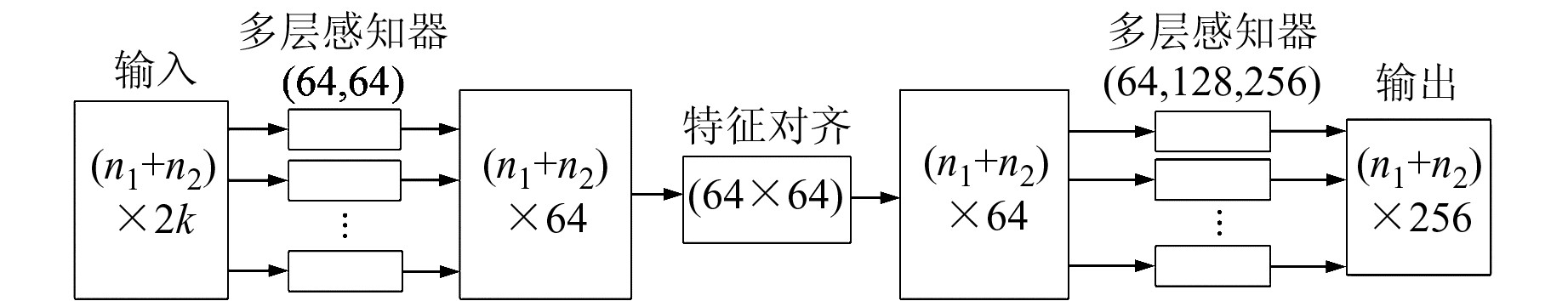图 2 改进的PointNet架构图 Fig. 2 Architecture diagram of improved PointNet

1.3 k-d树加速的ICP精配准

 $f\left( {{\mathit{\boldsymbol{R}}^k}, {\mathit{\boldsymbol{T}}^k}} \right) = \frac{1}{N}\mathop \sum \limits_{i = 1}^N {\left\| {{{\mathit{\boldsymbol{\overline a}} }_i} - {\mathit{\boldsymbol{R}}^k}\mathit{\boldsymbol{\overline b}} _i^{k - 1} - {\mathit{\boldsymbol{T}}^k}} \right\|^2}$ (8)

2 实验与分析 2.1 实验数据及环境

2.2 Lucy数据粗配准实验

Lucy数据实验仅测试粗配准效果，将从2个方面对该组数据的配准结果进行评价：1)模型在不同噪声水平下的配准均方根误差(root mean square error, RMSE)；2)与传统配准方法的效率和精度的比较。均方根误差通过查询源点云各点至目标点云中最近点获得，计算公式为：

 ${\mathop{\rm RMSE}\nolimits} = {\left( {\frac{1}{{{N_p}}}\sum\limits_{i = 1}^{{N_p}} {\left\| {\mathit{\boldsymbol{R}}{\mathit{\boldsymbol{p}}_i} + \mathit{\boldsymbol{T}} - {\mathit{\boldsymbol{q}}_i}} \right\|_2^2} } \right)^{\frac{1}{2}}}$ (9)

Lucy模型原始数据点云个数为1 300万，通过曲率压缩后为20万，再通过ISS检测出9 166个关键点，在此数据基础上随机删除1/100、1/50并进行刚性变换，添加递增的高斯白噪声以分成多组数据，得到的待配准点云个数分别为9 075和8 983。由图 3(a)可知，本文算法在随机删除1/100和1/50点的情况下，添加1~20 dB强度噪声时均能完成配准，且精度均在2.5 mm以内，噪声增强及缺失点数增多均会使配准误差变大。采用本文算法选择不同邻域点数计算法向量夹角及欧氏距离时，最终配准误差会产生约0.1 mm的差异，当点数为9 075和8 983时，选取20个邻域点的配准效果较好(图 3(b))。从表 1表 2可以看出，在Lucy数据中添加不同强度噪声时，本文算法平均配准时间比FPFH减少61.1 s，提高73.2%，比SHOT算法减少53.3 s，提高70.4%；平均配准精度比FPFH算法提高89.9%，比SHOT算法提高89.9%。图 4(a)为原始点云，4(b)、4(c)、4(d)为本文算法、FPFH算法、SHOT算法对Lucy模型添加1 dB噪声并删除1/50点的配准效果。从图中可以看出，本文算法配准后的点云重叠度优于FPFH、SHOT算法。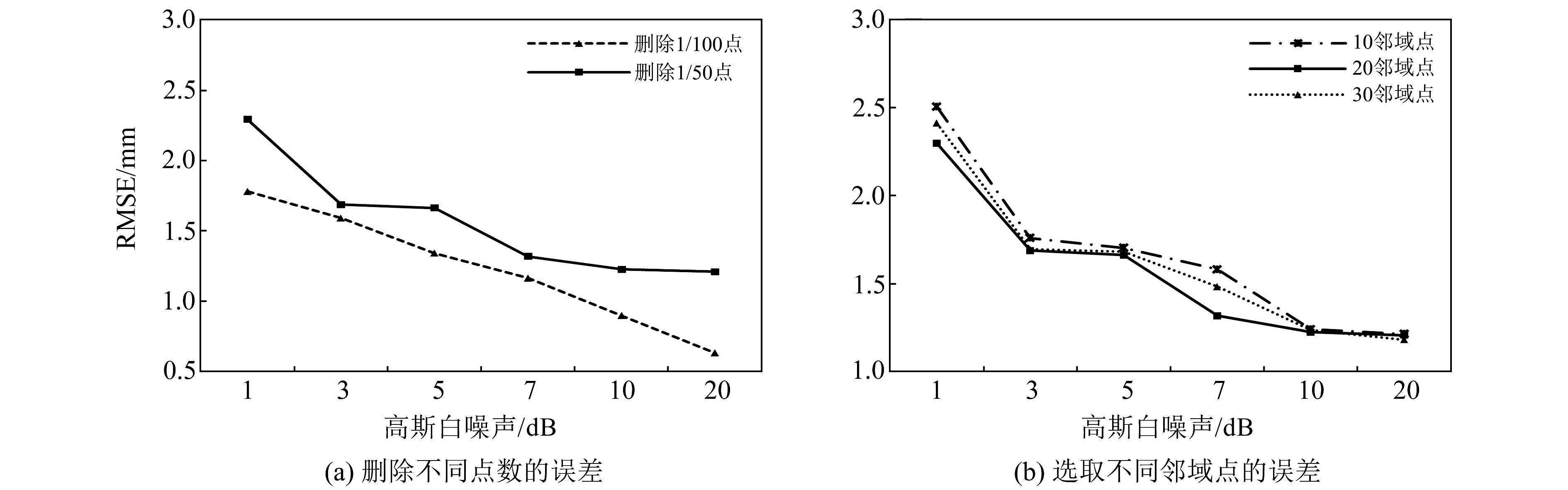图 3 多组实验配准误差对比图 Fig. 3 Comparison of registration error of several experiments表 1 不同噪声配准误差对比 Tab. 1 Comparison of registration error of different noise表 2 不同噪声配准时间对比 Tab. 2 Comparison of registration time of different noise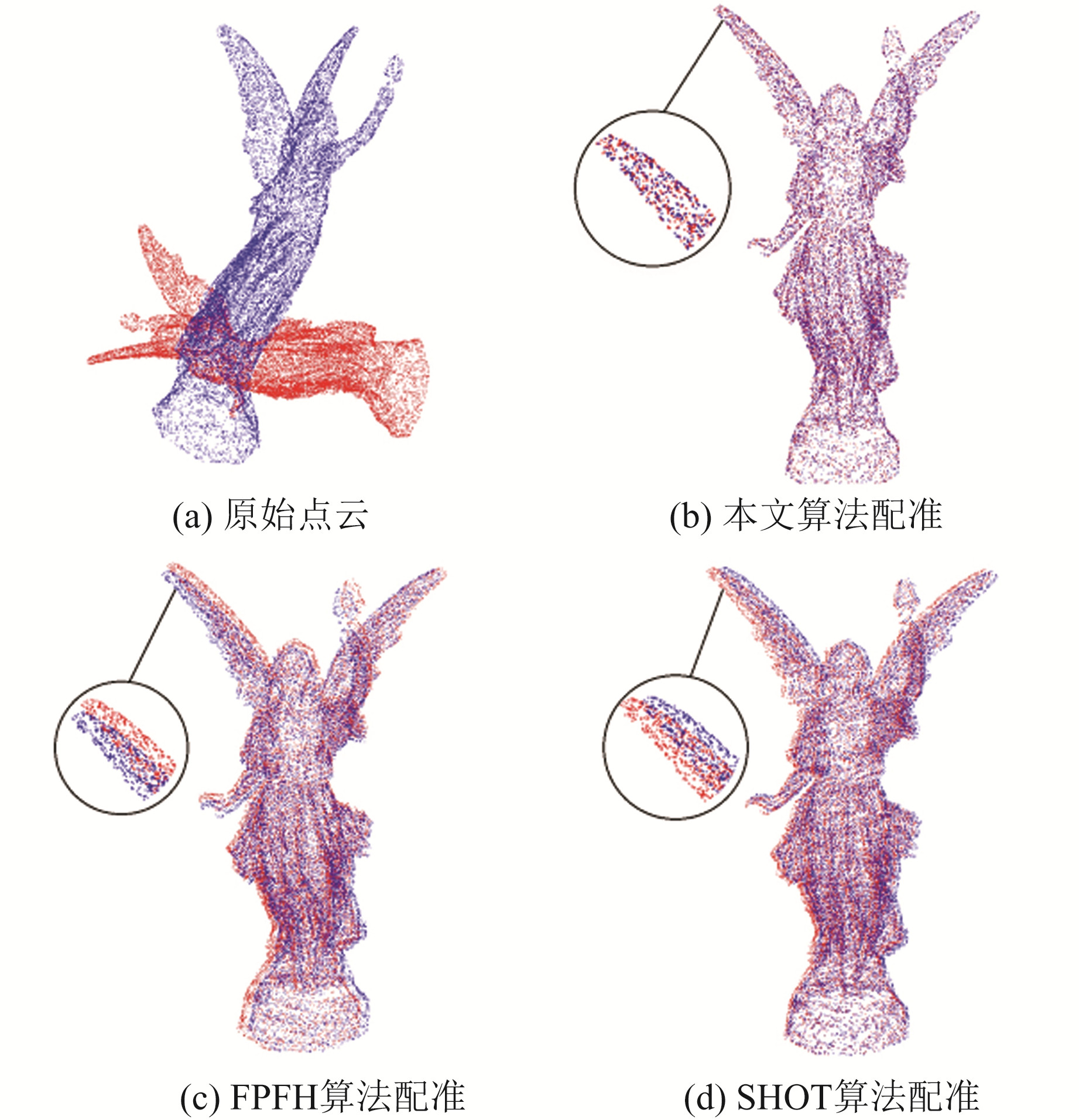图 4 Lucy配准图 Fig. 4 Registration diagrams of Lucy
2.3 Han数据精配准实验

Han两个站点曲率压缩和去噪后均约为20万点云，经ISS特征检测得到关键点数分别为11 249和11 890，站点间重叠度约为70%，计算PPF信息时选择20个邻域点。从图 5(a)5(b)可以看出，本文算法粗配准相比于FPFH算法能够提供更好的初始状态，以满足后续精配准的需求；图 5(c)5(d)为FPFH算法与本文算法在ICP精配准后的效果，两者在视觉上无明显区别。由表 3可知，本文算法配准过程相比于FPFH与ICP算法，运行时间减少100.5 s，提高68.6%，RMSE减小3.588 2 mm，提高28.1%，具有一定的优势。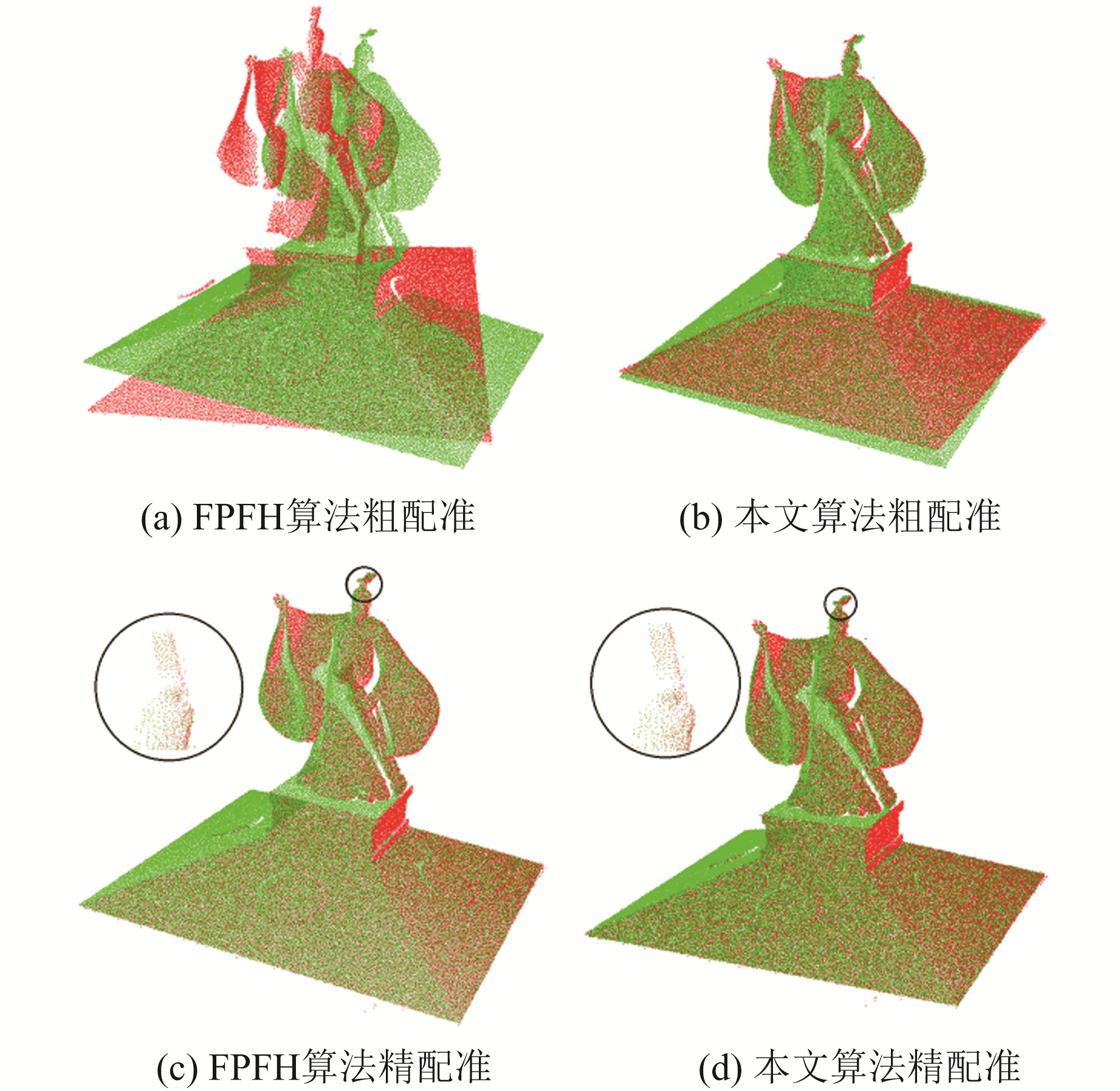图 5 Han配准图 Fig. 5 Registration diagrams of Han表 3 Han配准时间及误差对比 Tab. 3 Comparison of registration time and error of Han
2.4 Street数据精配准实验

Street两个站点曲率压缩和去噪后均约为30万点云，经ISS特征检测得到关键点数分别为9 005和8 512，站点间重叠度约为80%，计算PPF信息时选择20个邻域点。从图 6(b)可以看出，ISS关键点的分布位置在边缘处较为集中，能够较好地表达场景特征。从图 6(c)6(d)可以看出，本文算法的粗配准效果优于FPFH算法，能保持较好的初始配准姿态。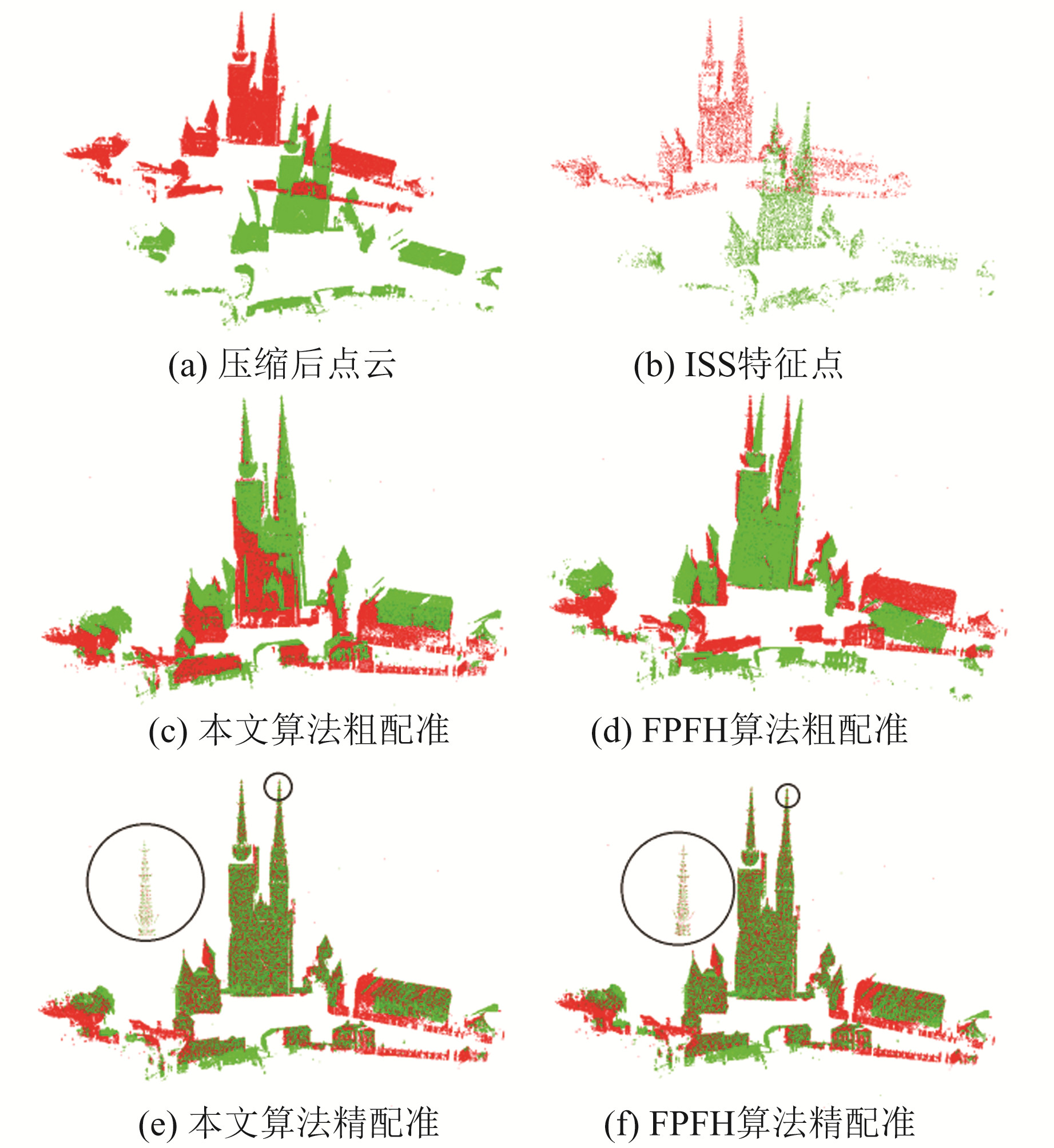图 6 Street配准图 Fig. 6 Registration diagrams of Street表 4 Street配准时间及误差对比 Tab. 4 Comparison of registration time and error of Street
3 结语

  Besl P J, McKay H D. A Method for Registration of 3-D Shapes[J]. IEEE Transactions on Pattern Analysis and Machine Intelligence, 1992, 14(2): 239-256 DOI:10.1109/34.121791 (0)  Yang J L, Li H D, Campbell D, et al. Go-ICP: A Globally Optimal Solution to 3D ICP Point-Set Registration[J]. IEEE Transactions on Pattern Analysis and Machine Intelligence, 2016, 38(11): 2 241-2 254 DOI:10.1109/TPAMI.2015.2513405 (0)  张崇军, 许烨璋, 郑善喜, 等. 改进权重的迭代最近点算法在点云配准中的应用[J]. 大地测量与地球动力学, 2019, 39(4): 417-420 (Zhang Chongjun, Xu Yezhang, Zheng Shanxi, et al. Improved Weight Iterative Closet Point Algorithm Applied in Point Cloud Registration[J]. Journal of Geodesy and Geodynamics, 2019, 39(4): 417-420) (0)  Charles R Q, Su H, Kaichun M, et al. PointNet: Deep Learning on Point Sets for 3D Classification and Segmentation[C]. 2017 IEEE Conference on Computer Vision and Pattern Recognition, Honolulu, 2017 (0)  Deng H W, Birdal T, Ilic S, et al. PPFNet: Global Context Aware Local Features for Robust 3D Point Matching[C]. 2018 IEEE/CVF Conference on Computer Vision and Pattern Recognition, Salt Lake City, 2018 (0)  白静, 司庆龙, 秦飞巍. 轻量级实时点云分类网络LightPointNet[J]. 计算机辅助设计与图形学学报, 2019, 31(4): 612-621 (Bai Jing, Si Qinglong, Qin Feiwei. Lightweight Real-Time Point Cloud Classification Network LightPointNet[J]. Journal of Computer-Aided Design and Graphics, 2019, 31(4): 612-621) (0)
Laser LiDAR Point Cloud Registration Algorithm Based on Feature Space Matching
CHEN Qiang1YUE Dongjie1     CHEN Jian1
1. School of Earth Science and Engineering, Hohai University, 8 West-Focheng Road, Nanjing 211100, China
Abstract: Aiming at the shortcomings of traditional feature-based coarse registration with low efficiency and many mismatches, we propose a registration method based on feature space matching. We extract the feature space using a simplified PointNet model. We take optimized point cloud PPF information as input and calculate the Euclidean distance according to the extracted feature space vector to filter out matching points. We eliminate the mismatched points to complete the coarse registration through RANSAC, and use ICP to realize fine registration. The results show that the proposed algorithm combined with ICP greatly improves the registration efficiency compared with FPFH and SHOT algorithm, and RMSE of the registration result is smaller.
Key words: 3D scanning; point cloud registration; PointNet model; random sample consensus; iterative closest point algorithm